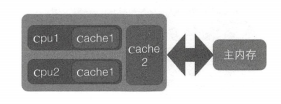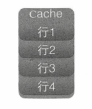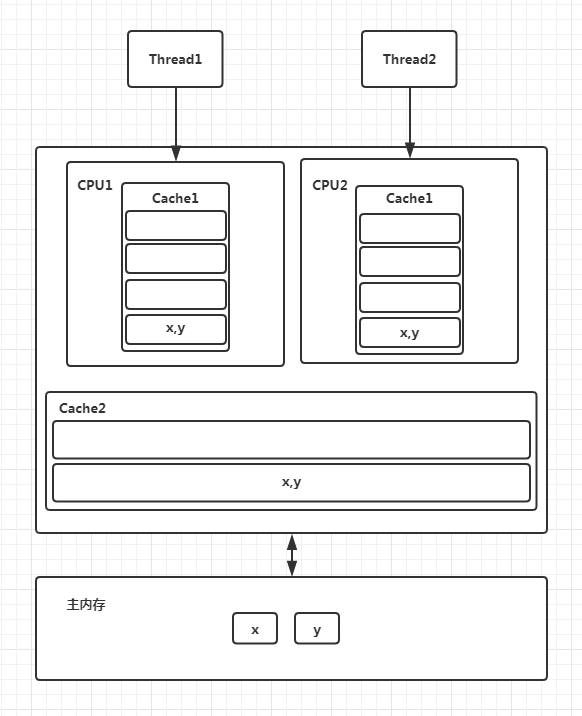# 什么是伪共享# 为何会出现伪共享

``````long a;
long b;
long c;
long d;
``````

``````public class ForContentTest {

static final int LINE_NUM = 1024;
static final int COLUMN_NUM = 1024;

public static void main(String[] args) {
long[][] array = new long[LINE_NUM][COLUMN_NUM];

long startTime = System.currentTimeMillis();
for (int i = 0; i < array.length; i++) {
for (int j = 0; j < array[i].length; j++) {
array[i][j] = i * 2 + j;
}
}

long endTime = System.currentTimeMillis();
long cacheTime = endTime - startTime;
System.out.println("cache time: " + cacheTime);
}

}
``````
``````cache time: 5
``````

``````public class ForContentTest2 {

static final int LINE_NUM = 1024;
static final int COLUMN_NUM = 1024;

public static void main(String[] args) {
long[][] array = new long[LINE_NUM][COLUMN_NUM];

long startTime = System.currentTimeMillis();
for (int i = 0; i < array.length; i++) {
for (int j = 0; j < array[i].length; j++) {
array[j][i] = i * 2 + j;
}
}

long endTime = System.currentTimeMillis();
long cacheTime = endTime - startTime;
System.out.println("not cache time: " + cacheTime);
}

}
``````
``````not cache time: 10
``````

# 如何避免伪共享

## JDK8之前

``````public final static class FilledLong {
public volatile long value = OL;
public long pl,p2,p3,p4,p5,p6;
}
``````

## JDK8

JDK 8提供了一个sun.misc.Contended 注解，用来解决伪共享问题。

### 修饰类

``````@sun.misc.Contended
public final static class FilledLong {
public volatile long value = OL;
}
``````

### 修饰变量

``````/** The current seed for a ThreadLocalRandom */
@sun.misc.Contended("tlr")

/** Probe hash value; nonzero if threadLocalRandomSeed initialized */
@sun.misc.Contended("tlr")

/** Secondary seed isolated from public ThreadLocalRandom sequence */
@sun.misc.Contended("tlr")